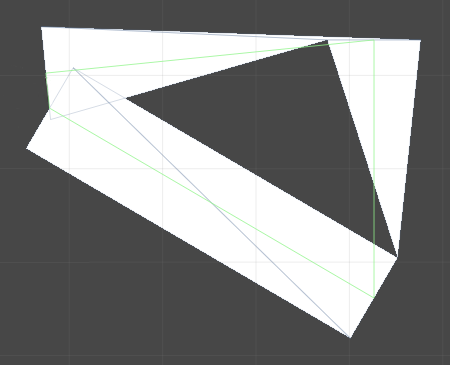# LineRenderer creating triangles instead of lines

Hi all,
I am having 2 problems drawing a line between four points.

1.) my line is not drawing correctly (some parts appear as triangles)…2.) when i flip the parent object of the line renderer by multiplying the x value in the transform’s localScale by -1, i get an odd result… the one part of the line that is working as i expected (the lower section) appears empty and i just get more triangles in the other sections, instead of getting the mirror image of the original line…

[29806-screen+shot+2014-07-26+at+8.39.25+pm.png|29806]

my code so far:

``````using UnityEngine;
using System.Collections;

public class Enemy : MonoBehaviour {
public Transform point1;
public Transform point2;
public Transform point3;
public Transform point4;
private GameObject flashObject;
private LineRenderer flashLineRenderer;
public Color flashColour = Color.white;

void Start ()
{
flashObject = new GameObject("FlashObject");
flashLineRenderer.material = flashLineMaterial;
flashLineRenderer.SetVertexCount(4);
flashLineRenderer.SetWidth(1f, 1f);
flashLineRenderer.SetPosition(0, point1.position);
flashLineRenderer.SetPosition(1, point2.position);
flashLineRenderer.SetPosition(2, point3.position);
flashLineRenderer.SetPosition(3, point4.position);
flashLineRenderer.useWorldSpace = false;
flashLineRenderer.SetColors(flashColour, flashColour);
flashObject.transform.parent = this.gameObject.transform;

}

}
``````

it would be great to get a bit of insight into what is causing these problems.

I’m having the same problem with LineRenderer. The first segment renders a triangle, unless I look at the other side. From that perspective it’s a rectangle. The last segment is always a rectangle.

I’ve played with linewidth etc, Here’s my code:

``````	lineRenderer.SetVertexCount (3);
lineRenderer.SetPosition (0, new Vector3(0.0f,0.0f,0.0f));
lineRenderer.SetPosition (1, new Vector3(5.0f,5.0f,5.0f));
lineRenderer.SetPosition (2, new Vector3(7.0f,2.0f,6.0f));
lineRenderer.SetWidth(0.1f,0.1f);
``````

Does anyone have an answer? Are there 2 sides to the line? It’s a billboard that always faces you.

One side renders a triangle, the other side renders a rectangle look at pics the underside pic.

Hi @Grin and @jbarbeau,

As you can read [here]. The problem is that a line render is a mesh and Unity optimize the mesh by reusing some vertex. The result is that ugly triangle shape.

You can solve this by

1. Creating more points to smooth the edges. Its not an elegant solution but its simple and works, at least for me. I wrote a function that do that:

Vector3 Generate_Points(Vector3 keyPoints, int segments=100){
Vector3 Points = new Vector3[(keyPoints.Length - 1) * segments + keyPoints.Length];
for(int i = 1; i < keyPoints.Length;i++){
Points [(i - 1) * segments + i - 1] = new Vector3(keyPoints [i-1].x,keyPoints [i-1].y,0);
for (int j = 1;j<=segments;j++){
float x = keyPoints [i - 1].x;
float y = keyPoints [i - 1].y;
float z = 0;//keyPoints [i - 1].z;
float dx = (keyPoints .x - keyPoints [i - 1].x)/segments;
_ float dy = (keyPoints .y - keyPoints [i - 1].y)/segments;_
_ Points [(i - 1) * segments + j + i - 1] = new Vector3 (x+dxj,y+dyj,z);
* }*
* }*
Points [(keyPoints.Length - 1) * segments + keyPoints.Length - 1] = new Vector3(keyPoints [keyPoints.Length-1].x,keyPoints [keyPoints.Length-1].y,0);_

* return Points;*
* }*
2. (Solution copied form [here])Coding your own line renderer, and not make it so economical. You can do this by generating a dynamic mesh. The mesh will consist of a series of thin quads. For each line segment, you can compute the four corners of the quad by calculating the normal of the line and a specified line width:
Vector3 normal = Vector3.Cross(start, end);
Vector3 side = Vector3.Cross(normal, end-start);
side.Normalize();
Vector3 a = start + side * (lineWidth / 2);
Vector3 b = start + side * (lineWidth / -2);
Vector3 c = end + side * (lineWidth / 2);
Vector3 d = end + side * (lineWidth / -2);
_: c# - How to make Line Renderer lines stay flat? - Game Development Stack Exchange
_
: c# - How to make Line Renderer lines stay flat? - Game Development Stack Exchange

If you don’t need the line to be blocky, you can use the Chaikin path smoothing algorithm http://graphics.cs.ucdavis.edu/education/CAGDNotes/Chaikins-Algorithm/Chaikins-Algorithm.html
It will produce a nicely smooth curve.